# Unit conversion - 6th grade (11y) - math problems

#### Number of problems found: 325

• The Nürburgring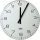The Nürburgring in Germany is a racetrack that hosts racing events all year. Two of the fastest laps ever driven on the track are 6 minutes 47.30 seconds and 6 minutes 52.01 seconds. How much faster is the 6 minutes 47.30 seconds time than the 6 minutes 5
• Clock losing time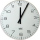A clock is showing 9:30 am. If the clock is 5 minutes slow, what would have been the correct time?
• A straight angle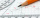A straight angle measures s, so you can subtract or add 85 from What are the measure of their angles?
• Cups of paintI have a board that is 2 meters wide and 3 meters tall. If one cup of paint cover 1,000 square centimeters. How many cups of paints does I need?
• Each ofEach of Suzan's notebooks is 4/5  inches wide. If she has 25 inches of space remaining on her bookshelf, how many notebooks will fit?
• Grocery storeYou spend 4/5 of an hour at the mall. You spend 1/3 of that time in the food court. How many minutes are you in the food court?
• Little 2Little fields farm lies on a rectangular plot of land that measures 2 2/4 miles in length and 2 2/3 miles in width. What is it's area in square miles?
• A rope 2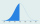A rope that is 6 meters long will be cut into 24 pieces that are all of the same length. What will be the length of each piece in centimeters? (100 cm = 1 m)
• A raindrop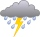A raindrop falls at a rate of 9.3 meters per second (m/s). Use the following facts to convert this speed to kilometers per hour (km/h). 1 km = 1000 m 1 min = 60 sec 1 hour = 60 min
• The physicalThe physical therapist asks Mr. Smith to walk 20 minutes in one hour to improve his ambulation. What fractional part of an hour is Mr. Smith to exercise?
• Tina has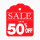Tina has 10 packages of yarn to knit a scarf. She uses 1/3 of package of yarn for each inch of length she adds to the scarf. If Tina uses all the yarn, how long will her scarf be?
• Bathtub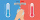Kevin has a bathtub shaped like a block. The bathtub measures 70 cm long, 45 cm wide and 50 cm high. Then the bathtub will be filled with clean water 3/5 of its height. The clean water needed is….
• The homeowner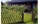The homeowner plans to build a fence along the sides and back of the property. How many feet of fencing are required to build the fence? (1 metre = 3.2808399 feet)Lots: 14w x 36.6d (m)
• Thomas 2Thomas is 56 3/4 inches tall. Jillian is 1 3/8 inches shorter than Thomas and Jane is 1 1/5 inches shorter than Jillian. How tall is Jane? Label the names on the tape diagram to represent this problem. Jane Jillian Thomas Excellent!
• Edges of the cuboid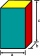Find the length of the edges of the cuboid, which has the following dimensions: width is 0.4 m; the height is 5.8 dm and the block can hold 81.2 liters of fluid.
• Her goal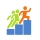Her goal is to walk 10,000 steps each day. She measures her average step length to be 30 inches. If she walks 10,000 steps in a day, how many miles will she walk?
• Water tank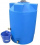The block-shaped tank is 2.5 m long, 100 cm wide and 12 dm high. In how many minutes will it be filled with water to two thirds if 40 liters of water flow into the tank per minute?
• Empty aquariumHow much does an empty aquarium weigh with dimensions: length = 40 cm, width = 30 cm, height = 20 cm, if 1 dm2 of glass weighs 300 g? Calculate its weight in kilograms.
• Electric wire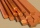William has a piece of wire that measures 1 yard long. He will use wire to fix several electrical outlets in his house. How many inches long is the wire? Solve this problem using bar diagrams.
• Minute handWhat is the distance the minute hand of the clock travels in 12 minutes, if the diameter of the clock is 30 cm and the hand extends to a distance of 2 cm from the edge of the clock?

Do you have an exciting math question or word problem that you can't solve? Ask a question or post a math problem, and we can try to solve it.

We will send a solution to your e-mail address. Solved examples are also published here. Please enter the e-mail correctly and check whether you don't have a full mailbox.

Unit conversion - math problems. Examples for 6th grade (the sixth graders).